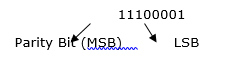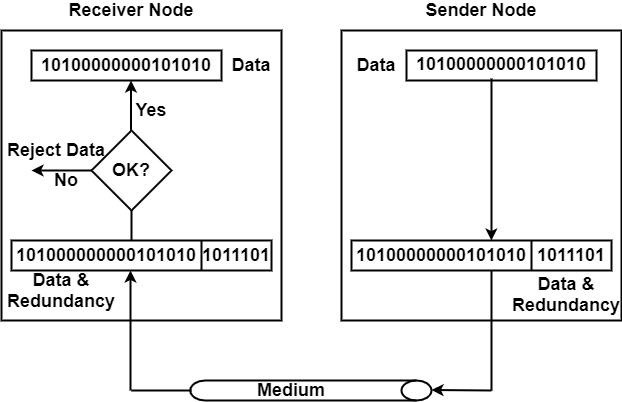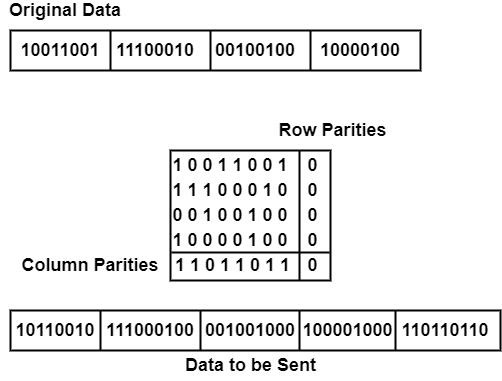# What is Parity Bit?

Parity Bit is the simplest and frequently used method for detecting an error. In this method, we have to join a parity bit to the end of the data structure. The bit is so chosen that the weight of codeword is either even or odd of, i.e. V is the cost of this bit is chosen therefore that the character has an even number of 1's called even parity or an odd number of 1's known as odd parity.

For Example, If the transmitter is transmitting 1110001 and using odd parity. It willappend 1 in sorting. The receiver determines the received character. Suppose the total number of 1's is odd. It considers that no error has appeared, and if one bit (any odd number of bits) is poorly inverted during communication, e.g. 11100001, the receiver will disclose an error.

## Techniques

There are two techniques used in Parity Bit are as follows −

### Simple Parity check

The structure of data from the source is inflicted to a check bit or parity bit generator structure, where parity of −

• 1 is inserted to the block if it includes an odd number of 1's, and

• 0 is added if it has an even number of 1's

This scheme creates a total number of 1's, even known as even parity checking.### Two-dimensional Parity check

Parity check bits are evaluated for each row, similar to a simple parity check bit. It is also calculated for all columns, and then both are transmitted along with the data. At the receiving end, these are distinguished with the parity bits evaluated on the received information.